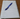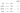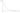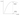Reading Notes - Ch. 6 - Fastbook

Multi-label Classification

When a classifier is being used in practice, it may encounter images that don't have any of the classes it was trained to classify. On the other hand, a single image may have multiple classes in it.

Multi-label classifiers are capable of classifying images that may not contain exactly one type of object. There may be no objects at all or even multiple objects.

Binary Cross Entropy

The cross-entropy loss function can be used to train a model to classify an image into one of several classes. It is not suitable for multi-label classification because:

• It tries to push one activation much ahead of others (due to the softmax function).
• It uses the negative log-likelihood which returns the loss value of just one activation.

The binary cross-entropy loss function allows for training a model that can classify an image into several classes. In essence, we treat each model output as an independent binary classifier that predicts whether a class is present in the image or not.

Let's assume that we want to build a model that can identify the following objects in an image:

1. Pen
2. Pencil
3. Eraser
4. Book

The model we build for this will have four outputs - one for each possible class.Consider the image below that has the objects pen and book.Let's assume the model's outputs are the following for this image:When using binary cross entropy, instead of using the softmax function which favors a single output over others, the model outputs are individually passed through a sigmoid activation function which squishes them between 0 and 1.We now need to push the outputs of the correct classes to 1 and the other outputs to 0.

We can replace the values corresponding to classes that don't exist in the image with 1 - value, so that we just have to focus on bringing all values as close to 1 as possible.Just like in cross-entropy loss, we take a log of the values to amplify small differences in values and then drop the negative sign (or equivalently, multiply by -1) so that the loss value is maximum when the value we calculated is close to 0. Conversely, the loss value is close to 0 when the value we calculated is close to 1.PyTorch provides two versions of the binary cross-entropy loss function that can be used:

1. BCELoss / F.binary_cross_entropy
2. BCEWithLogitsLoss / F.binary_cross_entropy_with_logits

The first assumes that you have passed the values you are feeding it through a sigmoid activation already. The second passes the values through a sigmoid before calculating the binary cross-entropy.Calculating performance

Since there isn't just one correct class, we can't just pick the output with the highest value as the model's prediction. Instead, we need to pick a threshold beyond which we'll consider the model output (after passing through a sigmoid activation) as 1, and values below this threshold will be considered 0.

If the threshold is too low, the model may pick incorrect classes. However, if the threshold is too high, the model will only pick classes for which it is very confident.

A good way to find the threshold is getting the raw model outputs (passed through sigmoid activation) and plot accuracy against different threshold values. We can then pick the threshold at which the accuracy is maximum.Regression

In a regression problem, the value we are trying to predict is one or more floats (continuous numeric value), for example: the center of a person's face in an image which is given by two values: the row and the column of the center of the face.

A loss function that can be used in regression problems is the MSELoss - mean squared error loss.